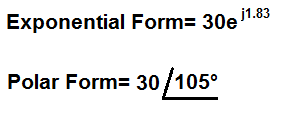﻿ Exponential to Polar Form Conversion Calculator ﻿# Exponential to Polar Form Conversion Calculator

FormulaExampleEnter the Amplitude (r) Enter the Phase (θ) radians

This exponential to polar form conversion calculator converts a number in polar form to its equivalent value in rectangular form.

Exponential forms of numbers take on the format, re, where r is the amplitude of the expression and θ is the phase of the expression. The amplitude r must be expressed in absolute value form. R cannot be a negative number. θ (theta) must be expressed in unit radians. Unlike the polar form, which is expressed in unit degrees, a complex exponential number is expressed in unit radians.

Polar, or phasor, forms of numbers take on the format, amplitude < phase. The phase is specified in degrees.

Exponential forms of numbers can be converted into their polar equivalents relatively easily. The amplitude of the exponential form is the direct amplitude of the polar form. So no conversion is necessary for the amplitude. The phase of the polar form must only be converted into its equivalent in degree value. So this is the only conversion that really needs to take place. Radians from the exponential form needs to be converted into degrees in the polar form.

So, for example, let's take the expression in exponential form, 20ej1.95. We want to convert this into its equivalent polar form. So, to do this, we take the formula and plug in the values, giving us, polar form= 20 < 111.73°.

And that's all that's required in order to convert from exponential form to polar form.

Usually, in electronics, polar forms are used in express components in AC circuit analysis. For example, if you are doing AC circuit analysis, one of the things that is required to do this is to convert the circuit from the time domain to the frequency domain, so that the circuit can be analyzed in the frequency domain. Therefore, the power source of the circuit is converted from the expression in the time domain to its expression in the frequency domain. In the frequency domain, the power source is expressed in polar form. So in situations like these, you need to convert values to their polar form.

Since the only 2 components of a number expressed in exponential form are the amplitude and the phase of the signal, these are the only 2 inputs required for the calculator to compute the equivalent number in polar form.

Related Resources

﻿